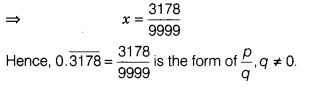# Express 0.3178 in the form of p/q .,q≠0

Express 0.3178 in the form of p/q .,q≠0

Let x = 0.3178
=f x = 0.317831783178 … (i)
=> 10000x = 3178.31783178 … (ii)
[multiplying 10000 both sides]
On subtracting Eq. (i) from Eq. (ii), we get
9999x = 3178